## Buildings.Utilities.Math

Library with functions such as for smoothing

### Information

This package contains blocks and functions for commonly used mathematical operations. The classes in this package augment the classes Modelica.Blocks.

Extends from Modelica.Icons.Package (Icon for standard packages).

### Package Content

Name DescriptionAverage Average of a vectorBesselJ0 Bessel function of the first kind of order 0, J0BesselJ1 Bessel function of the first kind of order 1, J1BesselY0 Bessel function of the second kind of order 0, Y0BesselY1 Bessel function of the second kind of order 1, Y1Bicubic Bicubic functionBinomial Binomial functionBiquadratic Biquadratic functionBooleanReplicator Boolean signal replicatorExponentialIntegralE1 Exponential integral function, E1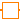Factorial Factorial functionFallingFactorial Falling factorial functionIntegerReplicator Integer signal replicatorIntegratorWithReset Output the integral of the input signalInverseXRegularized Function that approximates 1/x by a twice continuously differentiable functionMax Maximum element of a vectorMin Minimum element of a vectorPolynominal Polynominal functionPowerLinearized Power function that is linearized below a user-defined thresholdQuadraticLinear Function that is quadratic in first argument and linear in second argument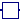RegNonZeroPower Power function, regularized near zero, but nonzero value for x=0SmoothExponential Once continuously differentiable approximation to exp(-|x|) in interval |x| < deltaSmoothHeaviside Twice continuously differentiable approximation to the Heaviside functionSmoothLimit Once continuously differentiable approximation to the limit functionSmoothMax Once continuously differentiable approximation to the maximum functionSmoothMin Once continuously differentiable approximation to the minimum functionSplice Block for splice function opertationTrapezoidalIntegration Integration using the trapezoidal ruleFunctions Package with mathematical functionsExamples Collection of models that illustrate model use and test models

##Buildings.Utilities.Math.Average

Average of a vector### Information

This block outputs the average of the vector.

Extends from Modelica.Blocks.Interfaces.MISO (Multiple Input Single Output continuous control block).

### Parameters

TypeNameDefaultDescription
Integernin1Number of inputs

### Connectors

TypeNameDescription
input RealInputu[nin]Connector of Real input signals
output RealOutputyConnector of Real output signal

### Modelica definition

block Average "Average of a vector" extends Modelica.Blocks.Interfaces.MISO; equation y = Buildings.Utilities.Math.Functions.average(u=u, nin=nin); end Average;

##Buildings.Utilities.Math.BesselJ0

Bessel function of the first kind of order 0, J0### Information

This block computes the bessel function of the first kind of order 0, J0.

Extends from Modelica.Blocks.Interfaces.SISO (Single Input Single Output continuous control block).

### Connectors

TypeNameDescription
input RealInputuConnector of Real input signal
output RealOutputyConnector of Real output signal

### Modelica definition

block BesselJ0 "Bessel function of the first kind of order 0, J0" extends Modelica.Blocks.Interfaces.SISO; equation y = Buildings.Utilities.Math.Functions.besselJ0(x=u); end BesselJ0;

##Buildings.Utilities.Math.BesselJ1

Bessel function of the first kind of order 1, J1### Information

This block computes the bessel function of the first kind of order 1, J1.

Extends from Modelica.Blocks.Interfaces.SISO (Single Input Single Output continuous control block).

### Connectors

TypeNameDescription
input RealInputuConnector of Real input signal
output RealOutputyConnector of Real output signal

### Modelica definition

block BesselJ1 "Bessel function of the first kind of order 1, J1" extends Modelica.Blocks.Interfaces.SISO; equation y = Buildings.Utilities.Math.Functions.besselJ1(x=u); end BesselJ1;

##Buildings.Utilities.Math.BesselY0

Bessel function of the second kind of order 0, Y0### Information

This block computes the bessel function of the second kind of order 0, Y0.

Extends from Modelica.Blocks.Interfaces.SISO (Single Input Single Output continuous control block).

### Connectors

TypeNameDescription
input RealInputuConnector of Real input signal
output RealOutputyConnector of Real output signal

### Modelica definition

block BesselY0 "Bessel function of the second kind of order 0, Y0" extends Modelica.Blocks.Interfaces.SISO; equation y = Buildings.Utilities.Math.Functions.besselY0(x=u); end BesselY0;

##Buildings.Utilities.Math.BesselY1

Bessel function of the second kind of order 1, Y1### Information

This block computes the bessel function of the second kind of order 1, Y1.

Extends from Modelica.Blocks.Interfaces.SISO (Single Input Single Output continuous control block).

### Connectors

TypeNameDescription
input RealInputuConnector of Real input signal
output RealOutputyConnector of Real output signal

### Modelica definition

block BesselY1 "Bessel function of the second kind of order 1, Y1" extends Modelica.Blocks.Interfaces.SISO; equation y = Buildings.Utilities.Math.Functions.besselY1(x=u); end BesselY1;

##Buildings.Utilities.Math.Bicubic

Bicubic function### Information

This block computes

y = a1 + a2 x1 + a3 x12 + a4 x2 + a5 x22 + a6 x1 x2 + a7 x1^3 + a8 x2^3 + a9 x12 x2 + a10 x1 x22

Extends from Modelica.Blocks.Interfaces.SI2SO (2 Single Input / 1 Single Output continuous control block).

### Connectors

TypeNameDescription
input RealInputu1Connector of Real input signal 1
input RealInputu2Connector of Real input signal 2
output RealOutputyConnector of Real output signal

### Modelica definition

block Bicubic "Bicubic function" extends Modelica.Blocks.Interfaces.SI2SO; input Real a "Coefficients"; equation y = Buildings.Utilities.Math.Functions.bicubic(a=a, x1=u1, x2=u2); end Bicubic;

##Buildings.Utilities.Math.Binomial

Binomial function### Information

This block computes the binomial coefficient "n choose k".

Extends from Modelica.Blocks.Interfaces.IntegerSO (Single Integer Output continuous control block).

### Connectors

TypeNameDescription
output IntegerOutputyConnector of Integer output signal
input IntegerInputnSize of set
input IntegerInputkSize of subsets

### Modelica definition

block Binomial "Binomial function" extends Modelica.Blocks.Interfaces.IntegerSO; Modelica.Blocks.Interfaces.IntegerInput n "Size of set"; Modelica.Blocks.Interfaces.IntegerInput k "Size of subsets"; equation y = Buildings.Utilities.Math.Functions.binomial(n=n, k=k); end Binomial;

##Buildings.Utilities.Math.Biquadratic### Information

This block computes

y = a1 + a2 x1 + a3 x12 + a4 x2 + a5 x22 + a6 x1 x2

Extends from Modelica.Blocks.Interfaces.SI2SO (2 Single Input / 1 Single Output continuous control block).

### Parameters

TypeNameDefaultDescription
Reala Coefficients

### Connectors

TypeNameDescription
input RealInputu1Connector of Real input signal 1
input RealInputu2Connector of Real input signal 2
output RealOutputyConnector of Real output signal

##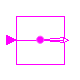Buildings.Utilities.Math.BooleanReplicator

Boolean signal replicator### Information

This block replicates the boolean input signal to an array of `nout` identical output signals.

Extends from Modelica.Blocks.Icons.BooleanBlock (Basic graphical layout of Boolean block).

### Parameters

TypeNameDefaultDescription
Integernout1Number of outputs

### Connectors

TypeNameDescription
input BooleanInputuConnector of boolean input signal
output BooleanOutputy[nout]Connector of boolean output signals

### Modelica definition

block BooleanReplicator "Boolean signal replicator" extends Modelica.Blocks.Icons.BooleanBlock; parameter Integer nout=1 "Number of outputs"; Modelica.Blocks.Interfaces.BooleanInput u "Connector of boolean input signal"; Modelica.Blocks.Interfaces.BooleanOutput y[nout] "Connector of boolean output signals"; equation y = Buildings.Utilities.Math.Functions.booleanReplicator(u=u, nout=nout); end BooleanReplicator;

##Buildings.Utilities.Math.ExponentialIntegralE1

Exponential integral function, E1### Information

This block computes the exponential integral, E1.

Extends from Modelica.Blocks.Interfaces.SISO (Single Input Single Output continuous control block).

### Connectors

TypeNameDescription
input RealInputuConnector of Real input signal
output RealOutputyConnector of Real output signal

### Modelica definition

block ExponentialIntegralE1 "Exponential integral function, E1" extends Modelica.Blocks.Interfaces.SISO; equation y = Buildings.Utilities.Math.Functions.exponentialIntegralE1(x=u); end ExponentialIntegralE1;

##Buildings.Utilities.Math.Factorial

Factorial function### Information

This block computes the factorial of the integer input, y=n!.

Extends from Modelica.Blocks.Interfaces.IntegerSO (Single Integer Output continuous control block).

### Connectors

TypeNameDescription
output IntegerOutputyConnector of Integer output signal
input IntegerInputuConnector of integer input signal

### Modelica definition

block Factorial "Factorial function" extends Modelica.Blocks.Interfaces.IntegerSO; Modelica.Blocks.Interfaces.IntegerInput u "Connector of integer input signal"; equation y = Buildings.Utilities.Math.Functions.factorial(n=u); end Factorial;

##Buildings.Utilities.Math.FallingFactorial

Falling factorial function### Information

This block computes the falling factorial, y = n.

Extends from Modelica.Blocks.Interfaces.IntegerSO (Single Integer Output continuous control block).

### Connectors

TypeNameDescription
output IntegerOutputyConnector of Integer output signal
input IntegerInputnInteger number
input IntegerInputkFalling factorial power

### Modelica definition

block FallingFactorial "Falling factorial function" extends Modelica.Blocks.Interfaces.IntegerSO; Modelica.Blocks.Interfaces.IntegerInput n "Integer number"; Modelica.Blocks.Interfaces.IntegerInput k "Falling factorial power"; equation y = Buildings.Utilities.Math.Functions.fallingFactorial(n=n, k=k); end FallingFactorial;

##Buildings.Utilities.Math.IntegerReplicator

Integer signal replicator### Information

This block replicates the integer input signal to an array of `nout` identical output signals.

Extends from Modelica.Blocks.Icons.IntegerBlock (Basic graphical layout of Integer block).

### Parameters

TypeNameDefaultDescription
Integernout1Number of outputs

### Connectors

TypeNameDescription
input IntegerInputuConnector of integer input signal
output IntegerOutputy[nout]Connector of integer output signals

### Modelica definition

block IntegerReplicator "Integer signal replicator" extends Modelica.Blocks.Icons.IntegerBlock; parameter Integer nout=1 "Number of outputs"; Modelica.Blocks.Interfaces.IntegerInput u "Connector of integer input signal"; Modelica.Blocks.Interfaces.IntegerOutput y[nout] "Connector of integer output signals"; equation y = Buildings.Utilities.Math.Functions.integerReplicator(u=u, nout=nout); end IntegerReplicator;

##Buildings.Utilities.Math.IntegratorWithReset

Output the integral of the input signal### Information

This model is similar to Modelica.Blocks.Continuous.Integrator except that it optionally allows to reset the output `y` of the integrator.

The output of the integrator can be reset as follows:

• If `reset = Buildings.Types.Reset.Disabled`, which is the default, then the integrator is never reset.
• If `reset = Buildings.Types.Reset.Parameter`, then a boolean input signal `trigger` is enabled. Whenever the value of this input changes from `false` to `true`, the integrator is reset by setting `y` to the value of the parameter `y_reset`.
• If `reset = Buildings.Types.Reset.Input`, then a boolean input signal `trigger` is enabled. Whenever the value of this input changes from `false` to `true`, the integrator is reset by setting `y` to the value of the input signal `y_reset_in`.

See Buildings.Utilities.Math.Examples.IntegratorWithReset for an example.

#### Implementation

To adjust the icon layer, the code of Modelica.Blocks.Continuous.Integrator has been copied into this model rather than extended.

Extends from Modelica.Blocks.Interfaces.SISO (Single Input Single Output continuous control block).

### Parameters

TypeNameDefaultDescription
Realk1Integrator gain 
ResetresetBuildings.Types.Reset.DisabledType of integrator reset
Initialization
RealOutputy.starty_startConnector of Real output signal
InitinitTypeModelica.Blocks.Types.Init.I...Type of initialization (1: no init, 2: steady state, 3,4: initial output)
Realy_start0Initial or guess value of output (= state)
Integrator reset
Realy_reset0Value to which integrator is reset, used if reset = Buildings.Types.Reset.Parameter

### Connectors

TypeNameDescription
input RealInputuConnector of Real input signal
input RealInputy_reset_inInput signal for state to which integrator is reset, enabled if reset = Buildings.Types.Reset.Input
input BooleanInputtriggerResets the integrator output when trigger becomes true

### Modelica definition

block IntegratorWithReset "Output the integral of the input signal" extends Modelica.Blocks.Interfaces.SISO(y(start=y_start)); parameter Real k(unit="1")=1 "Integrator gain"; /* InitialState is the default, because it was the default in Modelica 2.2 and therefore this setting is backward compatible */ parameter Modelica.Blocks.Types.Init initType=Modelica.Blocks.Types.Init.InitialState "Type of initialization (1: no init, 2: steady state, 3,4: initial output)"; parameter Real y_start=0 "Initial or guess value of output (= state)"; parameter Buildings.Types.Reset reset = Buildings.Types.Reset.Disabled "Type of integrator reset"; parameter Real y_reset = 0 "Value to which integrator is reset, used if reset = Buildings.Types.Reset.Parameter"; Modelica.Blocks.Interfaces.RealInput y_reset_in if reset == Buildings.Types.Reset.Input "Input signal for state to which integrator is reset, enabled if reset = Buildings.Types.Reset.Input"; Modelica.Blocks.Interfaces.BooleanInput trigger if reset <> Buildings.Types.Reset.Disabled "Resets the integrator output when trigger becomes true"; protected Modelica.Blocks.Interfaces.RealInput y_reset_internal "Internal connector for integrator reset"; Modelica.Blocks.Interfaces.BooleanInput trigger_internal "Needed to use conditional connector trigger"; initial equation if initType == Modelica.Blocks.Types.Init.SteadyState then der(y) = 0; elseif initType == Modelica.Blocks.Types.Init.InitialState or initType == Modelica.Blocks.Types.Init.InitialOutput then y = y_start; end if; equation der(y) = k*u; // Equations for integrator reset connect(trigger, trigger_internal); connect(y_reset_in, y_reset_internal); if reset <> Buildings.Types.Reset.Input then y_reset_internal = y_reset; end if; if reset == Buildings.Types.Reset.Disabled then trigger_internal = false; else when trigger_internal then reinit(y, y_reset_internal); end when; end if; end IntegratorWithReset;

##Buildings.Utilities.Math.InverseXRegularized

Function that approximates 1/x by a twice continuously differentiable function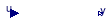### Information

Function that approximates y=1 ⁄ x inside the interval -δ ≤ x ≤ δ. The approximation is twice continuously differentiable with a bounded derivative on the whole real line.

See the package `Examples` for the graph.

Extends from Modelica.Blocks.Interfaces.SISO (Single Input Single Output continuous control block).

### Parameters

TypeNameDefaultDescription
Realdelta Abscissa value below which approximation occurs

### Connectors

TypeNameDescription
input RealInputuConnector of Real input signal
output RealOutputyConnector of Real output signal

### Modelica definition

block InverseXRegularized "Function that approximates 1/x by a twice continuously differentiable function" extends Modelica.Blocks.Interfaces.SISO; parameter Real delta(min=0) "Abscissa value below which approximation occurs"; equation y = Buildings.Utilities.Math.Functions.inverseXRegularized(x=u, delta=delta); end InverseXRegularized;

##Buildings.Utilities.Math.Max

Maximum element of a vector### Information

Outputs the maximum of the vector.

Extends from Modelica.Blocks.Interfaces.MISO (Multiple Input Single Output continuous control block).

### Parameters

TypeNameDefaultDescription
Integernin1Number of inputs

### Connectors

TypeNameDescription
input RealInputu[nin]Connector of Real input signals
output RealOutputyConnector of Real output signal

### Modelica definition

block Max "Maximum element of a vector" extends Modelica.Blocks.Interfaces.MISO; equation y = max(u); end Max;

##Buildings.Utilities.Math.Min

Minimum element of a vector### Information

Outputs the minimum of the vector.

Extends from Modelica.Blocks.Interfaces.MISO (Multiple Input Single Output continuous control block).

### Parameters

TypeNameDefaultDescription
Integernin1Number of inputs

### Connectors

TypeNameDescription
input RealInputu[nin]Connector of Real input signals
output RealOutputyConnector of Real output signal

### Modelica definition

block Min "Minimum element of a vector" extends Modelica.Blocks.Interfaces.MISO; equation y = min(u); end Min;

##Buildings.Utilities.Math.Polynominal

Polynominal function### Information

This block computes a polynomial of arbitrary order. The polynomial has the form

y = a1 + a2 x + a3 x2 + ...

Extends from Modelica.Blocks.Interfaces.SISO (Single Input Single Output continuous control block).

### Parameters

TypeNameDefaultDescription
Reala[:] Coefficients

### Connectors

TypeNameDescription
input RealInputuConnector of Real input signal
output RealOutputyConnector of Real output signal

### Modelica definition

block Polynominal "Polynominal function" extends Modelica.Blocks.Interfaces.SISO; parameter Real a[:] "Coefficients"; equation y = Buildings.Utilities.Math.Functions.polynomial(a=a, x=u); end Polynominal;

##Buildings.Utilities.Math.PowerLinearized

Power function that is linearized below a user-defined threshold### Information

Function that approximates y=xn where 0 < n so that
• the function is defined and monotone increasing for all x.
• dy/dx is bounded and continuous everywhere (for n < 1).

For x < x0, this function replaces y=xn by a linear function that is continuously differentiable everywhere.

A typical use of this function is to replace T = T4(1/4) in a radiation balance to ensure that the function is defined everywhere. This can help solving the initialization problem when a solver may be far from a solution and hence T4 < 0.

See the package `Examples` for the graph.

Extends from Modelica.Blocks.Interfaces.SISO (Single Input Single Output continuous control block).

### Parameters

TypeNameDefaultDescription
Realn Exponent
Realx0 Abscissa value below which linearization occurs

### Connectors

TypeNameDescription
input RealInputuConnector of Real input signal
output RealOutputyConnector of Real output signal

### Modelica definition

block PowerLinearized "Power function that is linearized below a user-defined threshold" extends Modelica.Blocks.Interfaces.SISO; parameter Real n "Exponent"; parameter Real x0 "Abscissa value below which linearization occurs"; equation y = Buildings.Utilities.Math.Functions.powerLinearized(x=u, n=n, x0=x0); end PowerLinearized;

##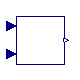Buildings.Utilities.Math.QuadraticLinear

Function that is quadratic in first argument and linear in second argument### Information

Block for function quadraticLinear, which computes

y = a1 + a2 x1 + a3 x12 + (a4 + a5 x1 + a6 x12) x2

Extends from Modelica.Blocks.Interfaces.SI2SO (2 Single Input / 1 Single Output continuous control block).

### Parameters

TypeNameDefaultDescription
Reala Coefficients

### Connectors

TypeNameDescription
input RealInputu1Connector of Real input signal 1
input RealInputu2Connector of Real input signal 2
output RealOutputyConnector of Real output signal

### Modelica definition

block QuadraticLinear "Function that is quadratic in first argument and linear in second argument" extends Modelica.Blocks.Interfaces.SI2SO; parameter Real a "Coefficients"; equation y = Buildings.Utilities.Math.Functions.quadraticLinear(a=a, x1=u1, x2=u2); end QuadraticLinear;

##Buildings.Utilities.Math.RegNonZeroPower

Power function, regularized near zero, but nonzero value for x=0### Information

Function that approximates y=|x|n where n > 0 so that
• y(0) is not equal to zero.
• dy/dx is bounded and continuous everywhere.

This function replaces y=|x|n in the interval -δ...+δ by a 4-th order polynomial that has the same function value and the first and second derivative at x=± δ.

A typical use of this function is to replace the function for the convective heat transfer coefficient for forced or free convection that is of the form h=c |dT|n for some constant c and exponent 0 ≤ n ≤ 1. By using this function, the original function that has an infinite derivative near zero and that takes on zero at the origin is replaced by a function with a bounded derivative and a non-zero value at the origin. Physically, the region -δ...+δ may be interpreted as the region where heat conduction dominates convection in the boundary layer.

See the package `Examples` for the graph.

Extends from Modelica.Blocks.Interfaces.SISO (Single Input Single Output continuous control block).

### Parameters

TypeNameDefaultDescription
Realn Exponent
Realdelta0.01Abscissa value where transition occurs

### Connectors

TypeNameDescription
input RealInputuConnector of Real input signal
output RealOutputyConnector of Real output signal

### Modelica definition

block RegNonZeroPower "Power function, regularized near zero, but nonzero value for x=0" extends Modelica.Blocks.Interfaces.SISO; parameter Real n "Exponent"; parameter Real delta = 0.01 "Abscissa value where transition occurs"; equation y = Buildings.Utilities.Math.Functions.regNonZeroPower(x=u, n=n, delta=delta); end RegNonZeroPower;

##Buildings.Utilities.Math.SmoothExponential

Once continuously differentiable approximation to exp(-|x|) in interval |x| < delta### Information

Function to provide a once continuously differentiable approximation to exp(- |x| ) in the interval |x| for some positive δ

Extends from Modelica.Blocks.Interfaces.SISO (Single Input Single Output continuous control block).

### Parameters

TypeNameDefaultDescription
Realdelta Transition point where approximation occurs

### Connectors

TypeNameDescription
input RealInputuConnector of Real input signal
output RealOutputyConnector of Real output signal

### Modelica definition

block SmoothExponential "Once continuously differentiable approximation to exp(-|x|) in interval |x| < delta" extends Modelica.Blocks.Interfaces.SISO; parameter Real delta "Transition point where approximation occurs"; equation y = Buildings.Utilities.Math.Functions.smoothExponential(x=u, delta=delta); end SmoothExponential;

##Buildings.Utilities.Math.SmoothHeaviside

Twice continuously differentiable approximation to the Heaviside function### Information

Twice Lipschitz continuously differentiable approximation to the Heaviside(.,.) function. See Example Buildings.Utilities.Math.Examples.SmoothHeaviside.

Extends from Modelica.Blocks.Interfaces.SISO (Single Input Single Output continuous control block).

### Parameters

TypeNameDefaultDescription
Realdelta Width of transition interval

### Connectors

TypeNameDescription
input RealInputuConnector of Real input signal
output RealOutputyConnector of Real output signal

### Modelica definition

block SmoothHeaviside "Twice continuously differentiable approximation to the Heaviside function" extends Modelica.Blocks.Interfaces.SISO; parameter Real delta(min=Modelica.Constants.eps) "Width of transition interval"; equation y = Buildings.Utilities.Math.Functions.smoothHeaviside(x=u, delta=delta); end SmoothHeaviside;

##Buildings.Utilities.Math.SmoothLimit

Once continuously differentiable approximation to the limit function### Information

Once continuously differentiable approximation to the limit(.,.) function. The output is bounded to be in [lower, upper].

Note that the limit need not be respected, such as illustrated in Buildings.Utilities.Math.Examples.SmoothMin.

Extends from Modelica.Blocks.Interfaces.SISO (Single Input Single Output continuous control block).

### Parameters

TypeNameDefaultDescription
RealdeltaX Width of transition interval
Realupper Upper limit
Reallower Lower limit

### Connectors

TypeNameDescription
input RealInputuConnector of Real input signal
output RealOutputyConnector of Real output signal

### Modelica definition

block SmoothLimit "Once continuously differentiable approximation to the limit function" extends Modelica.Blocks.Interfaces.SISO; parameter Real deltaX "Width of transition interval"; parameter Real upper "Upper limit"; parameter Real lower "Lower limit"; equation y = Buildings.Utilities.Math.Functions.smoothLimit(u, lower, upper, deltaX); end SmoothLimit;

##Buildings.Utilities.Math.SmoothMax

Once continuously differentiable approximation to the maximum function### Information

Once continuously differentiable approximation to the max(.,.) function.

Note that the maximum need not be respected, such as illustrated in Buildings.Utilities.Math.Examples.SmoothMin.

Extends from Modelica.Blocks.Interfaces.SI2SO (2 Single Input / 1 Single Output continuous control block).

### Parameters

TypeNameDefaultDescription
RealdeltaX Width of transition interval

### Connectors

TypeNameDescription
input RealInputu1Connector of Real input signal 1
input RealInputu2Connector of Real input signal 2
output RealOutputyConnector of Real output signal

### Modelica definition

block SmoothMax "Once continuously differentiable approximation to the maximum function" extends Modelica.Blocks.Interfaces.SI2SO; parameter Real deltaX "Width of transition interval"; equation y = Buildings.Utilities.Math.Functions.smoothMax(x1=u1, x2=u2, deltaX=deltaX); end SmoothMax;

##Buildings.Utilities.Math.SmoothMin

Once continuously differentiable approximation to the minimum function### Information

Once continuously differentiable approximation to the min(.,.) function.

Note that the minimum need not be respected, such as illustrated in Buildings.Utilities.Math.Examples.SmoothMin.

Extends from Modelica.Blocks.Interfaces.SI2SO (2 Single Input / 1 Single Output continuous control block).

### Parameters

TypeNameDefaultDescription
RealdeltaX Width of transition interval

### Connectors

TypeNameDescription
input RealInputu1Connector of Real input signal 1
input RealInputu2Connector of Real input signal 2
output RealOutputyConnector of Real output signal

### Modelica definition

block SmoothMin "Once continuously differentiable approximation to the minimum function" extends Modelica.Blocks.Interfaces.SI2SO; parameter Real deltaX "Width of transition interval"; equation y = Buildings.Utilities.Math.Functions.smoothMin(x1=u1, x2=u2, deltaX=deltaX); end SmoothMin;

##Buildings.Utilities.Math.Splice

Block for splice function opertation### Information

This block implements Buildings.Utilities.Math.Functions.spliceFunction, which provides a continuously differentiable transition between two arguments.

Extends from Modelica.Blocks.Icons.Block (Basic graphical layout of input/output block).

### Parameters

TypeNameDefaultDescription
Realdeltax Half width of transition interval

### Connectors

TypeNameDescription
input RealInputxIndependent value
input RealInputu1Argument of u > 0 (pos)
input RealInputu2Argument of u < 0 (neg)
output RealOutputySmoothed value

### Modelica definition

block Splice "Block for splice function opertation" extends Modelica.Blocks.Icons.Block; Modelica.Blocks.Interfaces.RealInput x "Independent value"; Modelica.Blocks.Interfaces.RealInput u1 "Argument of u > 0 (pos)"; Modelica.Blocks.Interfaces.RealInput u2 "Argument of u < 0 (neg)"; Modelica.Blocks.Interfaces.RealOutput y "Smoothed value"; parameter Real deltax "Half width of transition interval"; equation y=Buildings.Utilities.Math.Functions.spliceFunction( pos=u1, neg= u2, x= x, deltax= deltax); end Splice;

##Buildings.Utilities.Math.TrapezoidalIntegration

Integration using the trapezoidal rule### Information

This function computes a definite integral using the trapezoidal rule.

Extends from Modelica.Blocks.Interfaces.MISO (Multiple Input Single Output continuous control block).

### Parameters

TypeNameDefaultDescription
Integernin1Number of inputs
IntegerN Number of integrand points
RealdeltaX Width of interval for Trapezoidal integration

### Connectors

TypeNameDescription
input RealInputu[nin]Connector of Real input signals
output RealOutputyConnector of Real output signal

### Modelica definition

model TrapezoidalIntegration "Integration using the trapezoidal rule" extends Modelica.Blocks.Interfaces.MISO; parameter Integer N "Number of integrand points"; parameter Real deltaX "Width of interval for Trapezoidal integration"; equation y = Buildings.Utilities.Math.Functions.trapezoidalIntegration(N=N, f=u, deltaX=deltaX); end TrapezoidalIntegration;